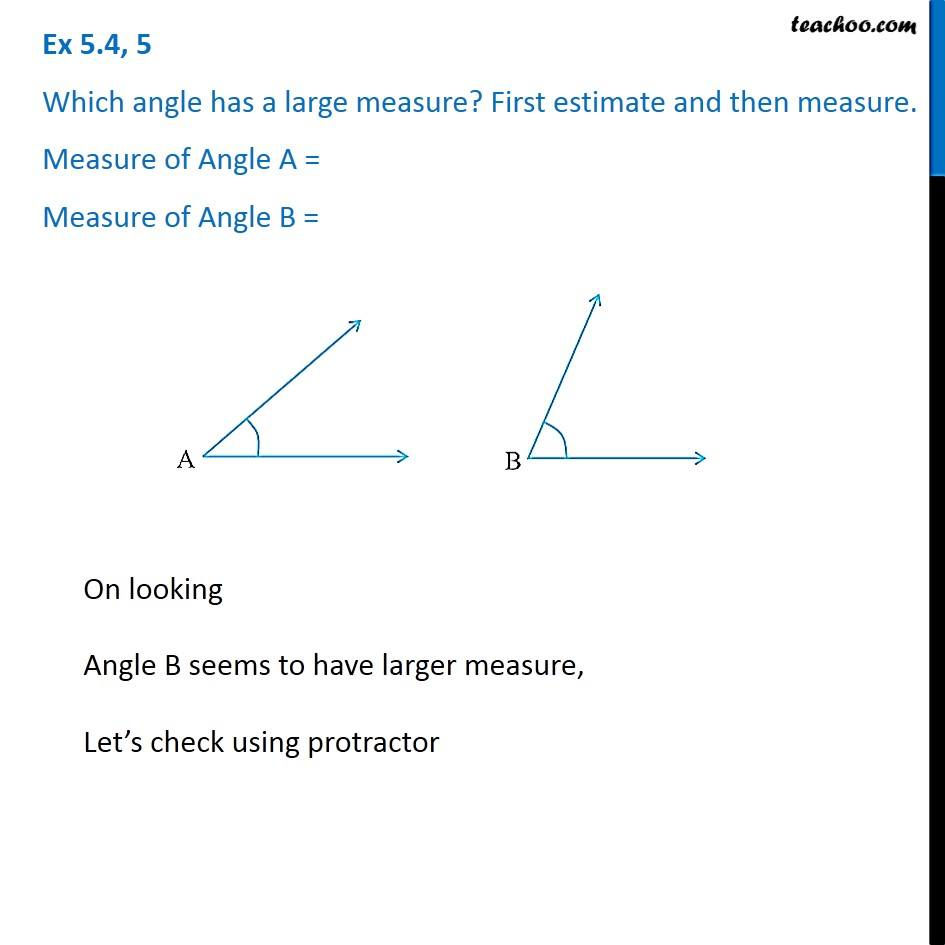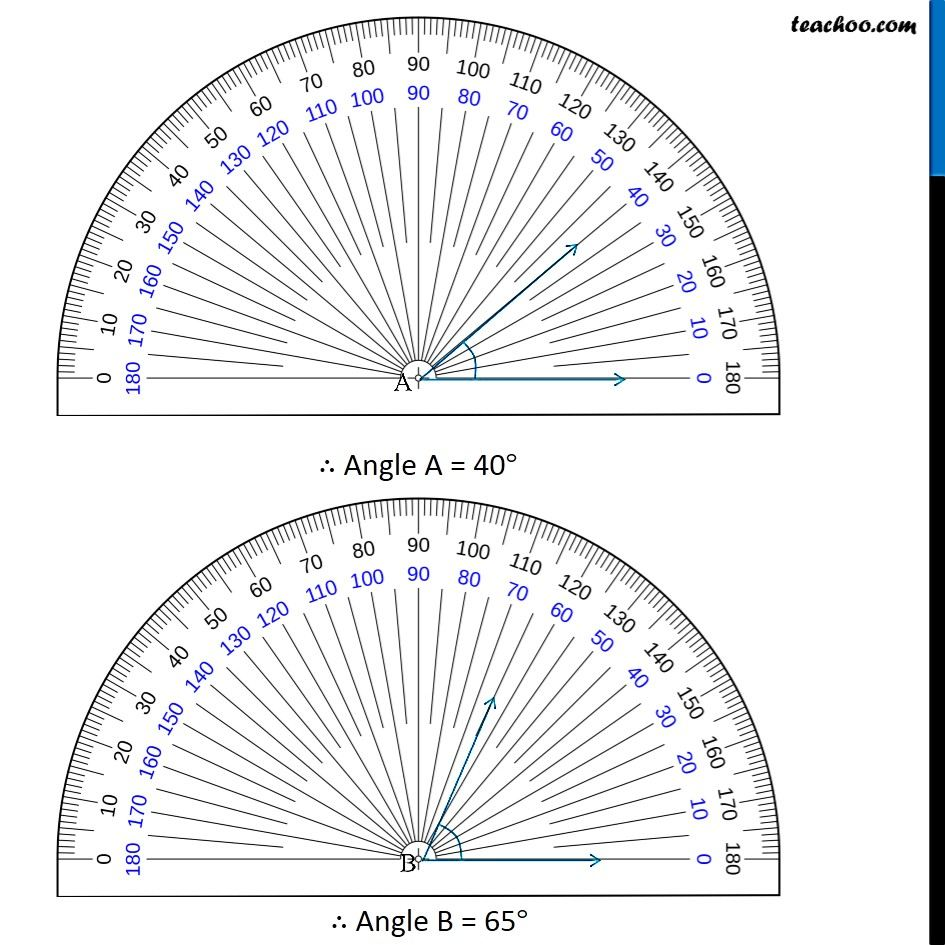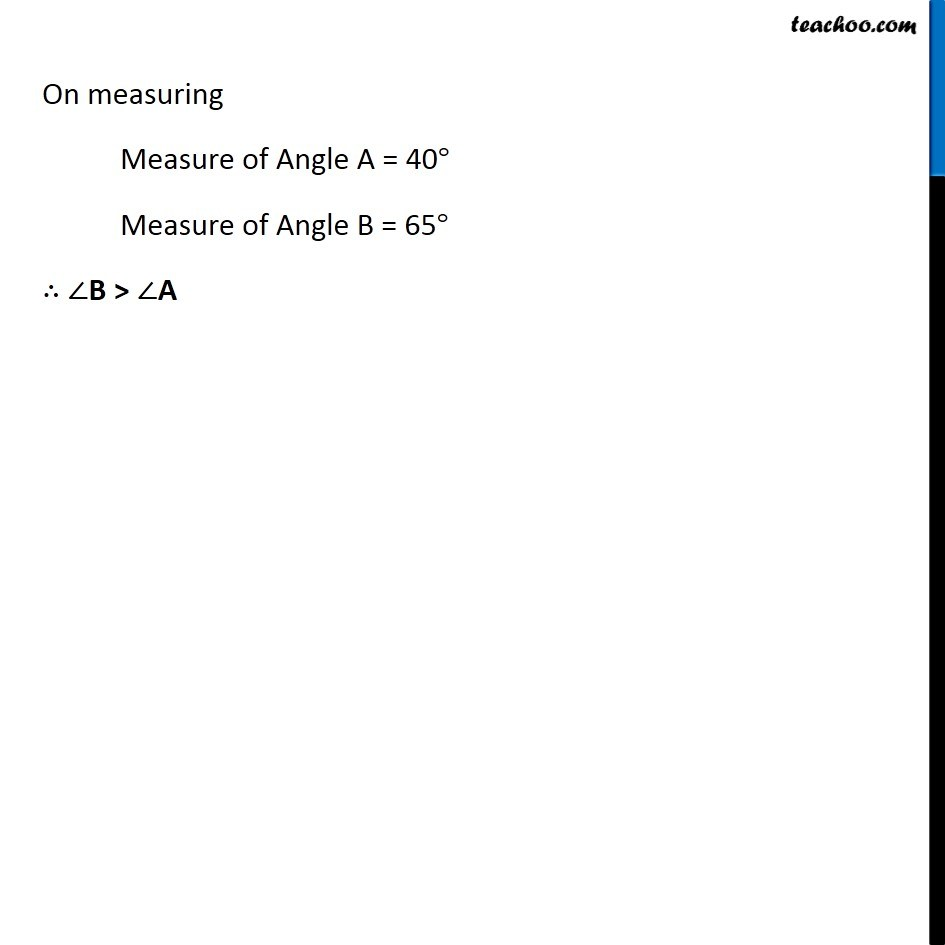Subscribe to our Youtube Channel - https://you.tube/teachoo

1. Chapter 5 Class 6 Understanding Elementary Shapes
2. Concept wise
3. Measuring angles using protractor

Transcript

Ex 5.4, 5 Which angle has a large measure? First estimate and then measure. Measure of Angle A = Measure of Angle B = On looking Angle B seems to have larger measure, Let’s check using protractor On measuring Measure of Angle A = 40° Measure of Angle B = 65° ∴ ∠B > ∠A

Measuring angles using protractor